This document contains R versions of the boxed examples from Chapter 11 of the “Analysis and Interpretation of Freshwater Fisheries Data” book. Some sections build on descriptions from previous sections, so each section may not stand completely on its own. More thorough discussions of the following items are available in linked vignettes:

The following additional packages are required to complete all of the examples (with the required functions noted as a comment and also noted in the specific examples below).

> library(FSA)          # Subset, fitPlot, ks2d2, ks2d2p, headtail
> library(car)          # Anova
> library(ggplot2)      # qplot, facet_grid
> library(Hmisc)        # rcorr
> library(lattice)      # xyplot
> library(MASS)         # manova
> library(quantreg)     # rq et al.

The G statistic used in Box 11.1 does not appear in base R or any package that I am aware of. However, Dr. Peter Hurd has provided code on his web page that performs this analysis. This function can be loaded into R as follows.

> source("http://www.psych.ualberta.ca/~phurd/cruft/g.test.r")

In addition, external tab-delimited text files are used to hold the data required for each example. These data are loaded into R in each example with read.table(). Before using read.table() the working directory of R must be set to where these files are located on your computer. The working directory for all data files on my computer is set with

> setwd("C:/aaaWork/Web/fishR/BookVignettes/aiffd2007")

In addition, I prefer to not show significance stars for hypothesis test output, reduce the margins on plots, alter the axis label positions, and reduce the axis tick length. In addition, contrasts are set in such a manner as to force R output to match SAS output for linear model summaries. All of these options are set with

> options(width=90,continue=" ",show.signif.stars=FALSE,
contrasts=c("contr.sum","contr.poly"))
> par(mar=c(3.5,3.5,1,1),mgp=c(2.1,0.4,0),tcl=-0.2)

## 11.1 Pooling Prey Items as a Single Resource

Prey items in fish stomachs can sometimes be pooled prior to analysis. To determine whether two (or more) prey items act as a single resource, we can use chi-square contingency table analysis. In the example below, we are interested in whether prey i and prey j can be pooled prior to analysis.

### 11.1.1 Preparing Data

These simple data can be entered directly into a matrix with matrix(). This function takes a vector of measurements as the first argument, the number of rows in the matrix in the nrow= argument, and whether or not the values should be placed in the matrix by rows and then columns or vice versa in the byrow= argument (byrow=TRUE enters the data by rows first). The columns and rows can then be labeled with colnames() and rownames(), respectively.

> d1 <- matrix(c(18,9,18,25),nrow=2,byrow=TRUE)
> colnames(d1) <- c("J.present","J.absent")
> rownames(d1) <- c("I.present","I.absent")
> d1
          J.present J.absent
I.present        18        9
I.absent         18       25
> addmargins(d1)                                 # for display only
          J.present J.absent Sum
I.present        18        9  27
I.absent         18       25  43
Sum              36       34  70

### 11.1.2 G-Test and Chi-Square Test

The g-test used in the box is computed by submitting the data matrix to g.test().

> ( g1 <- g.test(d1) )
Log likelihood ratio (G-test) test of independence without correction with d1
Log likelihood ratio statistic (G) = 4.1457, X-squared df = 1, p-value
= 0.04174

The G-test used above is an alternative to the more traditional chi-square test. The chi-square test is constructed using chisq.test() with the data matrix as the first argument and, optionally, turning off the default continuity correction with correct=FALSE.

> ( chi1 <- chisq.test(d1,correct=FALSE) )
Pearson's Chi-squared test with d1
X-squared = 4.0857, df = 1, p-value = 0.04325

### 11.1.3 Association with Cross-Product Ratio

The cross-product ratio to determine the direction of association is computed by extracting positions from the matrix as follows.

> (d1[1,1]*d1[2,2])/(d1[1,2]*d1[2,1])
 2.777778

## 11.2 Presenting Diet Measures Graphically

By combining different diet measures in two-dimensional space, graphical techniques can relay important information about feeding behavior of fishes. Using Figure 11.3 (in text), we can interpret feeding strategies of each predator population in the graphs presented (in text).

### 11.2.1 Preparing Data

The Box11_2.txt data file is read and the structure is observed. Note that the pred1 variable is a group factor variable that corresponds to the letters on the graphics in the box.

> d2 <- read.table("data/Box11_2.txt",header=TRUE)
> str(d2)
'data.frame':   36 obs. of  4 variables:
$freqocc : num 0.06 0.03 0.05 0.06 0.29 0.16 0.1 0.04 0.04 0.24 ...$ abundance: num  2.41 26.46 43.3 53.95 38.83 ...
$pred : Factor w/ 4 levels "charr80","charr89",..: 1 1 1 1 1 1 1 1 1 1 ...$ pred1    : Factor w/ 4 levels "A","B","C","D": 1 1 1 1 1 1 1 1 1 1 ...

### 11.2.2 Graphic – Lattice Approach

One of the easier ways to construct this graphic is to use xyplot() from the lattice package. This function requires a formula of the form y~x|factor as its first argument and the data frame containing those variables in the data= argument. The pch=19 argument is used to set the plotting character to solid circles. The layout=c(1,4) argument is used to force the panels to appear in four rows by one column. Finally, the as.table=TRUE argument forces the function to plot levels in order from the top of the graph rather than from the bottom.

> xyplot(abundance~freqocc|pred1,data=d2,pch=19,layout=c(1,4),as.table=TRUE,
xlab="Frequency of Occurrence",ylab="Prey-Specific Abundance")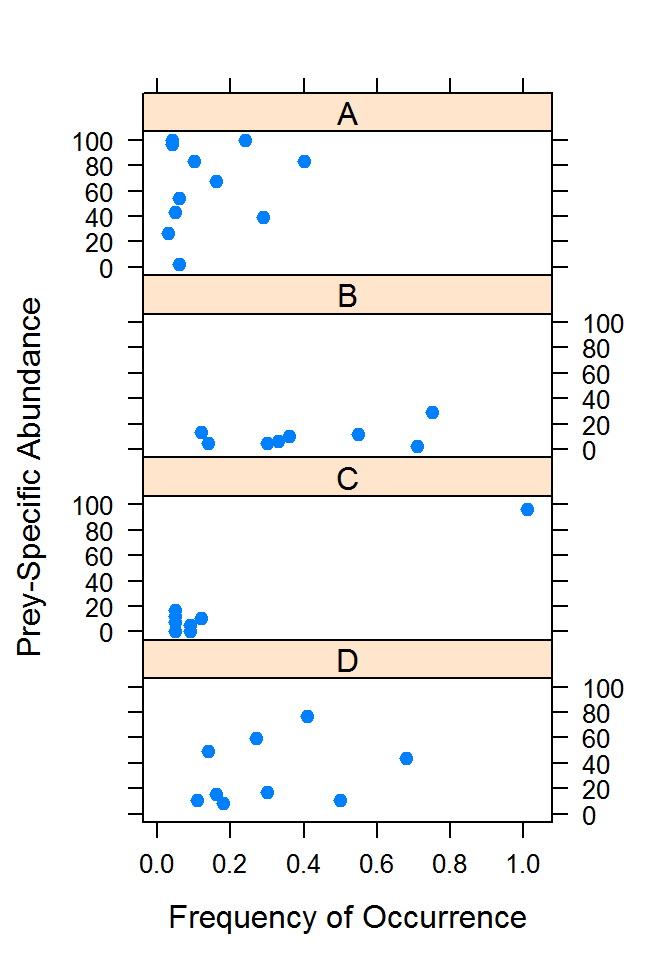### 11.2.3 Graphic – ggplot2 Approach

A similar graphic can be constructed using qplot() and facet_grid() from the ggplot2 package. The qplot() function requires the x and y variables as the first two arguments followed by a data= argument. The facet_grid() function requires a formula that describes the subgroups to be plotted in separate panels. As there is only one variable for the panels it must appear either on the left- or right-side of the tilde. If it is on the left-side then the panels are vertical and there must then be a “dot” on the right-side of the tilde.

> qplot(freqocc,abundance,data=d2,xlab="Frequency of Occurrence",
ylab="Prey-Specific Abundance") + facet_grid(pred1~.)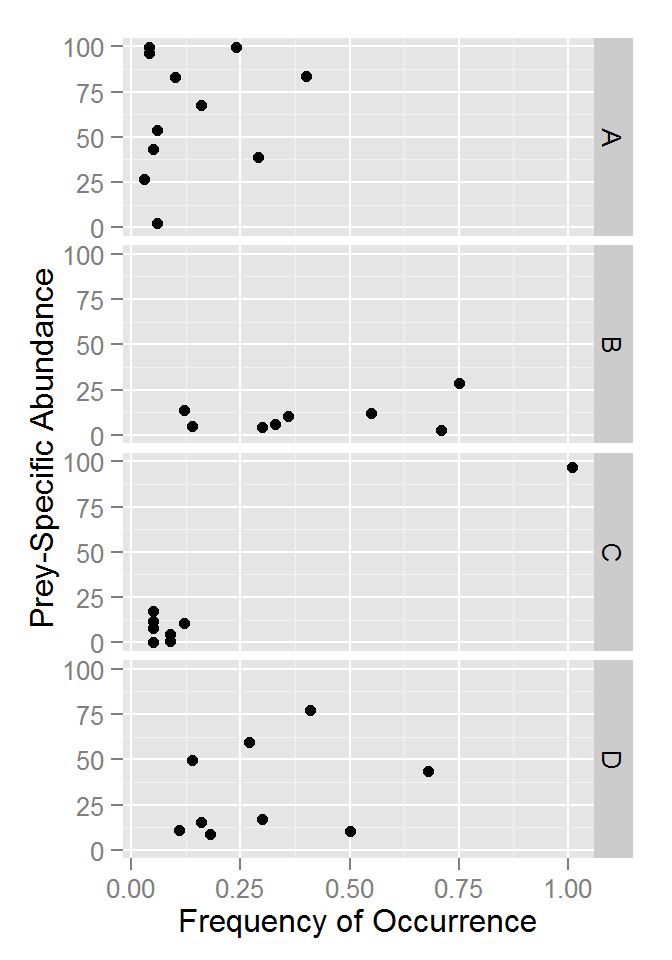## 11.3 Determining the Minimum and Maximum Sizes of Prey

The maximum size of prey in fish diets often increases with body size. However, the minimum size of prey may change relatively little. In addition to determining the mean or median size of prey consumed by use of bivariate plots, investigators may want to characterize the maximum and minimum sizes consumed (i.e., the edges of the scattergrams). Least absolute values regression (LAV), also called least absolute deviations regression, can be used to evaluate these types of diet data (Scharf et al. 1998). An extension of LAV, quantile regression, fits any specified quantile as a linear regression model. The LAV is the 50th percentile (median) in quantile regression. Such an analysis is available in the Blossom Statistical Software Package (Cade and Richards (2000)); available at http://www.mesc.usgs.gov). This program generates test statistics by permutations of the original data through re-randomization. The R code producing similar results to the Blossom package is listed below.

The quantile regression methodology used in this vignette is from the quantreg package. A vignette that details the use of this methodology in more detail can be found by typing vignette("rq",package="quantreg").

### 11.3.1 Preparing Data

The Box11_3.txt data file is read and the structure is observed.

> d3 <- read.table("data/Box11_3.txt",header=TRUE)
> str(d3)
'data.frame':   53 obs. of  2 variables:
$LMB.len : num 68 68 45 54 86 97 119 87 59 68 ...$ PREY.len: num  23.2 17.9 15.2 23.7 25.4 ...

### 11.3.2 Quick Exploration

A scatterplot of prey fish length on Largemouth Bass length is constructed with plot() using a formula of the form y~x, the usual data= argument, and optional labels for the x- and y-axes.

> plot(PREY.len~LMB.len,data=d3,pch=19,xlab="Largemouth Bass Length (mm)",
ylab="Prey Fish Length (mm)")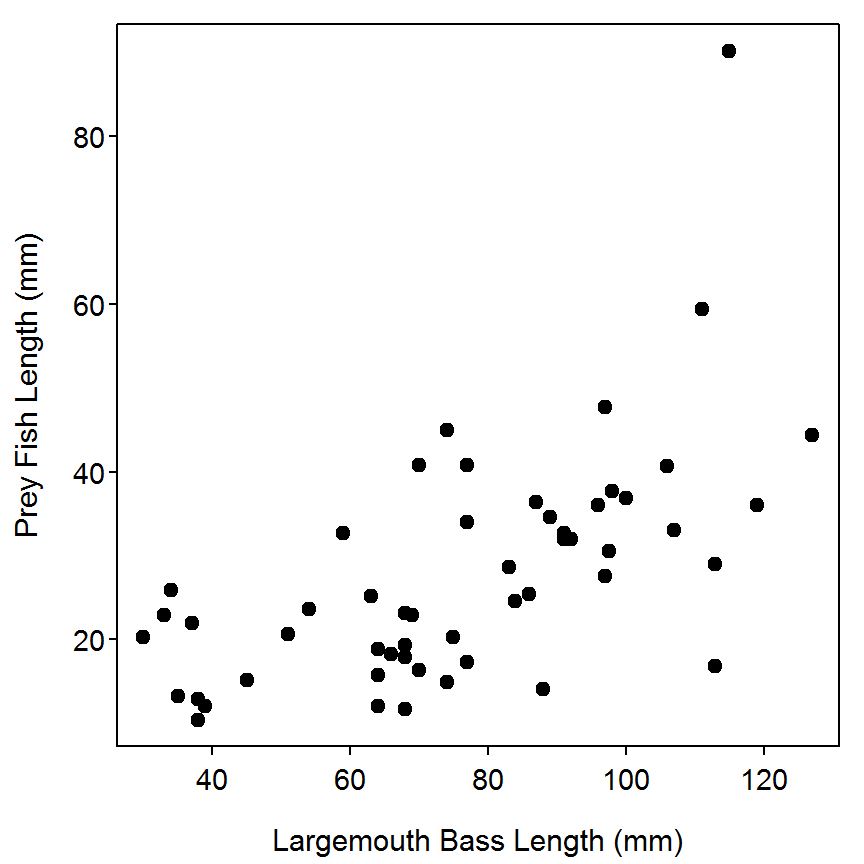### 11.3.3 Quantile Regression – Fitting a Specific Quantile

Quantile regressions are fit in R with rq() from the quantreg package. This function takes many of the same arguments at lm() – in particular, a formula of the form response~explanatory and the usual data= argument. However, very importantly, it has an additional argument, called tau=, which contains decimal representations of the quantiles to be fit. For example, to fit the median (i.e., 50th quantile) one would use tau=0.5. The estimated coefficients, and parametric confidence intervals, are extracted from the saved rq object with summary(). Typical inferential methods for the coefficient parameters can be seen by including the covariance=TRUE argument in summary(). Botstrap estimates of the standard error and p-value can be estimated by including the se="boot" argument in summary(). These results show that the median quantile regression has an intercept of -0.370 and a slope of 0.349 with the confidence interval bounds shown below. Additionally, the intercept of the median regression is not different than zero and that there is a significantly positive slope.

> qr1 <- rq(PREY.len~LMB.len,data=d3,tau=0.5)
> summary(qr1,covariance=TRUE)

Call: rq(formula = PREY.len ~ LMB.len, tau = 0.5, data = d3)

tau:  0.5

Coefficients:
Value    Std. Error t value  Pr(>|t|)
(Intercept) -0.37047  4.78248   -0.07746  0.93856
LMB.len      0.34870  0.05714    6.10286  0.00000
> summary(qr1,covariance=TRUE,se="boot")

Call: rq(formula = PREY.len ~ LMB.len, tau = 0.5, data = d3)

tau:  0.5

Coefficients:
Value    Std. Error t value  Pr(>|t|)
(Intercept) -0.37047  4.89729   -0.07565  0.94000
LMB.len      0.34870  0.06255    5.57511  0.00000

### 11.3.4 Quantile Regression – Fitting Many Quantiles

The tau= argument to rq() can take a vector of values such that a wide number of quantiles are fit simultaneously. For example, the code below fits and summarizes the 5th, 25th, 50th, 75th, and 95th quantile regressions. The coef() function can be used to extract just the model coefficients from the saved rq object.

> taus <- c(0.05,0.25,0.50,0.75,0.95)
> qr2 <- rq(PREY.len~LMB.len,data=d3,tau=taus)
> summary(qr2)

Call: rq(formula = PREY.len ~ LMB.len, tau = taus, data = d3)

tau:  0.05

Coefficients:
coefficients lower bd  upper bd
(Intercept)   5.96667    -33.73177   8.29816
LMB.len       0.09559      0.02036   0.32160

Call: rq(formula = PREY.len ~ LMB.len, tau = taus, data = d3)

tau:  0.25

Coefficients:
coefficients lower bd upper bd
(Intercept) -0.56737     -9.17489  4.73383
LMB.len      0.28935      0.18239  0.38235

Call: rq(formula = PREY.len ~ LMB.len, tau = taus, data = d3)

tau:  0.5

Coefficients:
coefficients lower bd upper bd
(Intercept) -0.37047     -2.70994 13.71577
LMB.len      0.34870      0.20301  0.38367

Call: rq(formula = PREY.len ~ LMB.len, tau = taus, data = d3)

tau:  0.75

Coefficients:
coefficients lower bd upper bd
(Intercept) 12.64381      2.23211 18.18596
LMB.len      0.25562      0.22817  0.45451

Call: rq(formula = PREY.len ~ LMB.len, tau = taus, data = d3)

tau:  0.95

Coefficients:
coefficients lower bd upper bd
(Intercept)  9.70914      4.25405 15.45307
LMB.len      0.47647      0.41189  0.82055
> coef(qr2)
             tau= 0.05  tau= 0.25  tau= 0.50  tau= 0.75 tau= 0.95
(Intercept) 5.96666551 -0.5673656 -0.3704704 12.6438069 9.7091354
LMB.len     0.09559163  0.2893529  0.3486976  0.2556231 0.4764703

A plot of the five quantile regression lines superimposed onto the observed data is obtained by simply plotting the raw data (as done above) and then using a abline() within a loop to superimpose the fitted quantile regression lines. This plot suggests that the near minimum prey length increases only slightly with increasing predator length (slope=0.096) whereas the near maximum prey length increases more dramatically (slope=0.476). The relationship in the middle of the distribution is a bit more difficult to interpret as the first quartile (quantile=0.25) and median regression show a fairly steep relationship whereas the third quartile (quantile=0.75) is fairly shallow. This result could be a function of the distributions of prey lengths available to the predators.

> plot(PREY.len~LMB.len,data=d3,pch=19,xlab="Largemouth Bass Length (mm)",
ylab="Prey Fish Length (mm)")
> for (i in 1:length(taus)) abline(coef=coef(qr2)[,i],col=gray(taus[i]),lwd=2)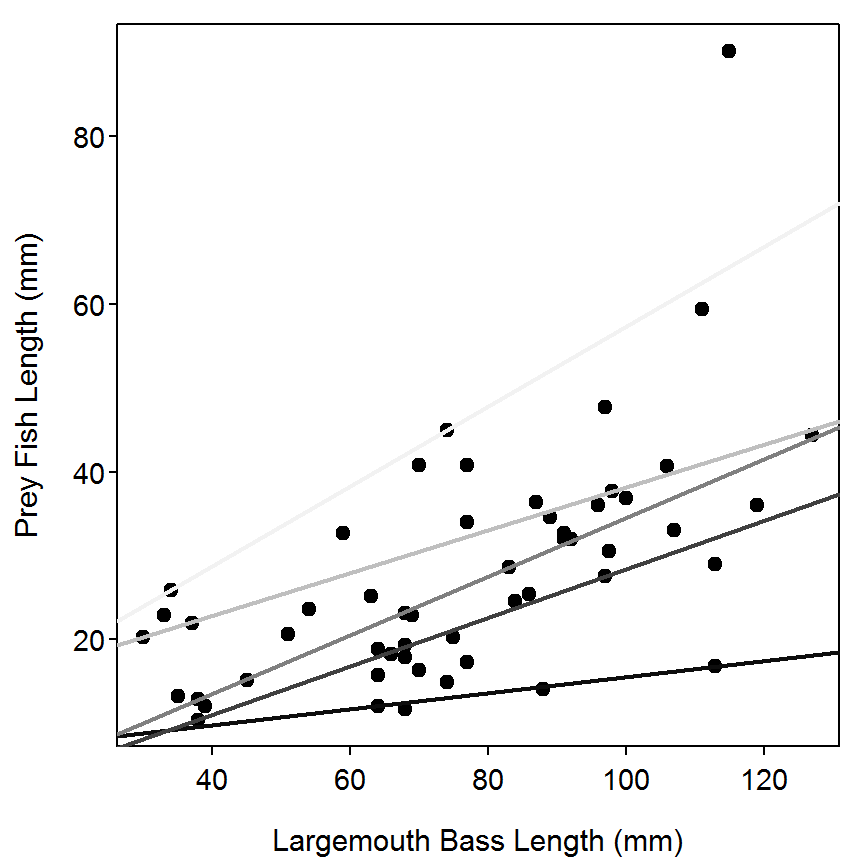The ability for the tau= argument to be a vector allows the fishery scientist to examine a wide variety of quantile regressions at once. For example, the code below computes the quantile regressions for all quantiles between 0.05 and 0.95 in steps of 0.01. The results of the fitting AND the summary function are saved to objects for later plotting using plot(). The plots below illustrate the intercept (top) and slope (bottom) estimates for each quantile. The results for the slope generally mirror what was observed above. In general, the relationship between prey and predator lengths increases fairly dramatically from the near minimum to approximately the median regression, the becomes more shallow until about the 80th percentile, before becoming much steeper to the near maximum values. Thus, it appears that approximately the length of the lower half and the upper fifth of prey consumed increases dramatically with increasing predator length but the the mid to upper lengths increase less strongly.

> taus <- (5:95)/100
> qr3 <- rq(PREY.len~LMB.len,data=d3,tau=taus)
> sqr3 <- summary(qr3)
> plot(qr3)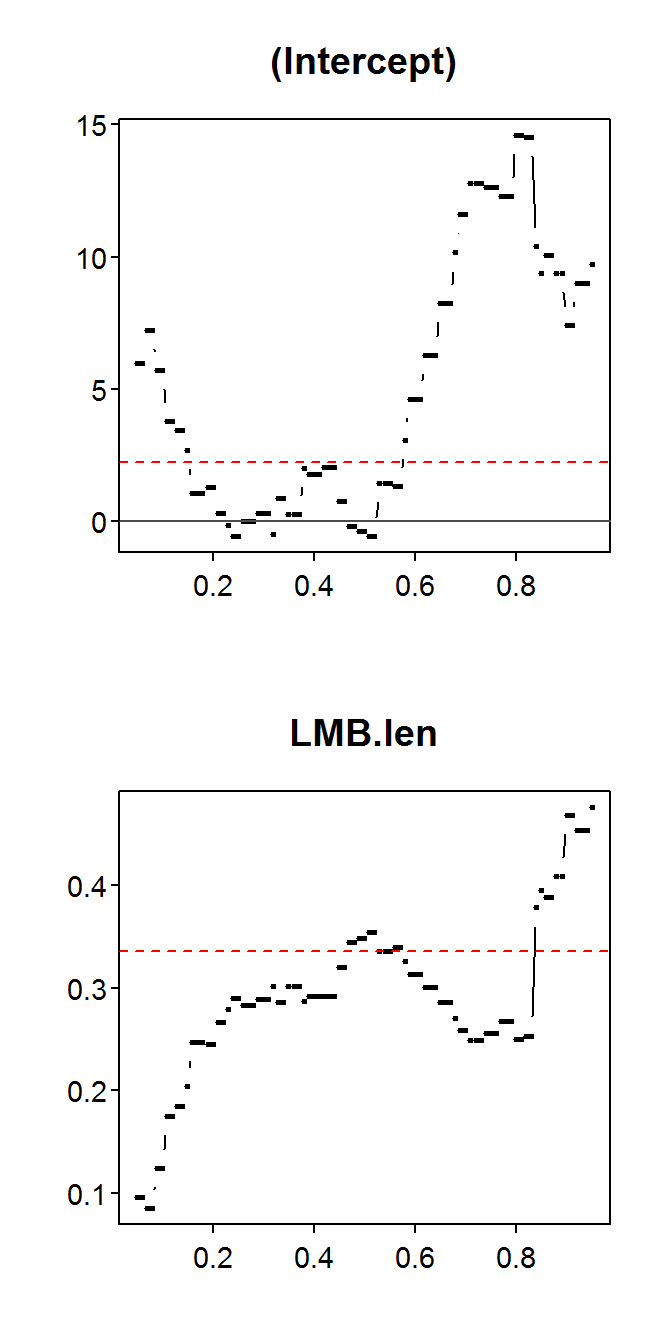The plots below are similar to the ones above except that 90% confidence bands have been added to the parameter estimates. The confidence bands for the slope illustrate a relatively poor fit (wide intervals) at the extreme quantiles, very left-skewed intervals from approximately 0.5 to 0.65 and very right-skewed intervals from approximately 0.65 to 0.85. These results indicate a lack of precision probably due to a relatively small sample size.

> plot(sqr3)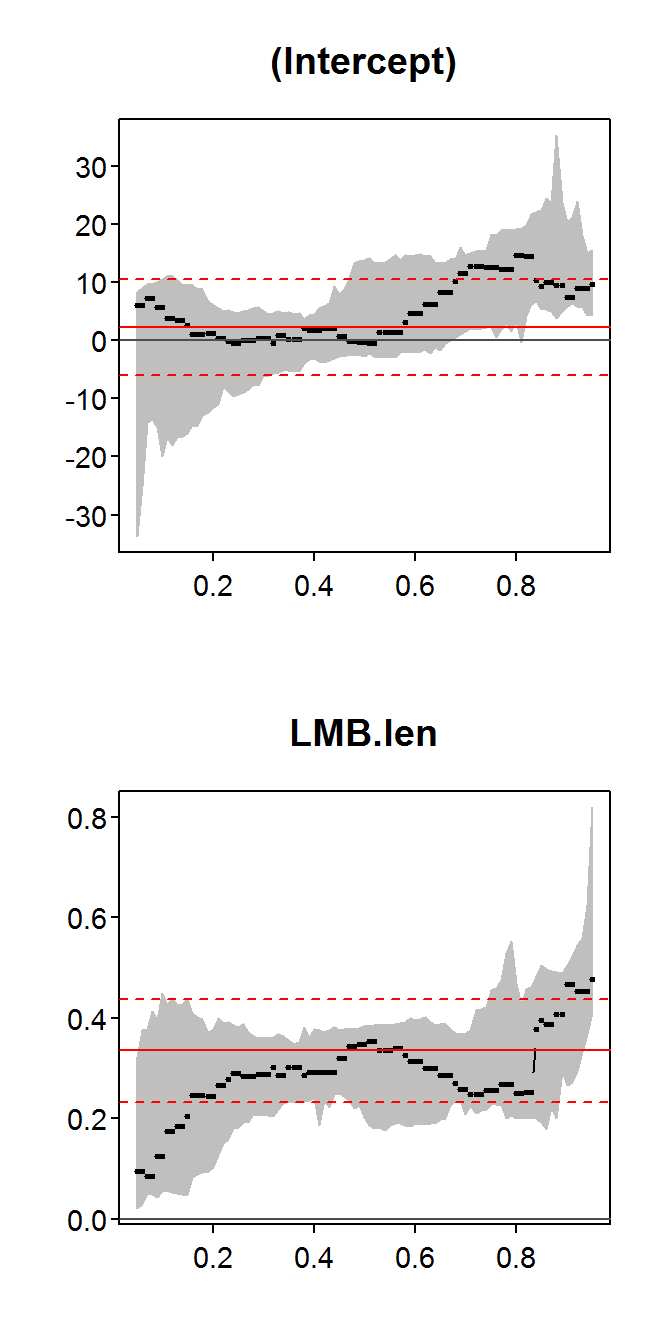## 11.4 Analyzing Diet Data with Repeated-Measures Analysis of Variance (ANOVA)

NOT YET COMPLETED

## 11.5 Assessing Spatial Patterns in Diet with the Two-Dimensional Kolmogorov-Smirnov Test

Several statistical methods are available to relate diet patterns to the distribution of habitat in aquatic systems. Mantel and partial-Mantel tests are powerful techniques that test whether spatial patterns are random or due to some treatment (or time). These tests are not specifically discussed here. More information can be obtained in Fortin and Gurevitch (1993) and Chapter 18 of AIFFD. If spatial data can be arranged into bivariate spatial coordinates, a two-dimensional Kolmogorov-Smirnov (2DKS) test can be used to (1) identify whether a single distribution has arisen by random effects or (2) compare two bivariate distributions (see Garvey et al. (1998) for a review). This nonparametric test finds the maximum difference, $$D_{bks}$$ (where $$bks$$ represents bivariate Kolmogorov-Smirnov), in integrated probabilities for four quadrants around each point in a plane. If the maximum $$D_{bks}$$ between two distributions exceeds that expected randomly, we conclude that they differ. The significance of the test statistic $$D_{bks}$$ is determined by rerandomizing the original data 5000 times and then comparing this randomly genereated distribution to the observed value.

In the following hypothetical example, we want to know how vegetation in a large lake affects piscivory in age-0 smallmouth bass (Micropterus dolomieu). We partition the bottom of a shallow lake into 80 habitat quadrants (20 x 4) and determine whether each contains vegetation. Within each quadrant, we sample smallmouth bass diets by means of gastric lavage and note whether piscivory is present or absent.

### 11.5.1 Preparing Data

The Box11_5.txt data file is read and the structure is observed. The main data frame is first split into two data frames based on the two scenarios using Subset() and each of those data frames is split into two data frames based on the site charachteristc variable. These splittings result in four data frames as defined by two scenarios (A and B) and two site characteristcs (fish present" and “vegetations present”).

> d5 <- read.table("data/Box11_5.txt",header=TRUE)
> str(d5)
'data.frame':   60 obs. of  4 variables:
$scenario : Factor w/ 2 levels "A","B": 1 1 1 1 1 1 1 1 1 1 ...$ site.char: Factor w/ 2 levels "fish.present",..: 1 1 1 1 1 1 1 1 1 1 ...
$coord1 : int 1 2 3 4 5 8 9 9 10 10 ...$ coord2   : int  1 2 1 2 1 4 3 4 3 4 ...
> #Split the data frame into the two scenarios
> d5A <- Subset(d5,scenario=="A")
> d5B <- Subset(d5,scenario=="B")
>
> #Split each scenario into the fish present and vegetation present distributions,
> d5Af <- Subset(d5A,site.char=="fish.present")
> d5Av <- Subset(d5A,site.char=="vegetation.present")
> d5Bf <- Subset(d5B,site.char=="fish.present")
> d5Bv <- Subset(d5B,site.char=="vegetation.present")

### 11.5.2 Plots

Plots for both scenarios with different symbols for the fish and vegetation present information are constructed by using plot() to plot the data for the vegetation and then using points() to add the data for fish distributions.

> plot(coord2~coord1,data=d5Av,pch=2,cex=1.5,xlab="Coordinate 1",ylab="Coordinate 2"
,xlim=c(1,20),ylim=c(1,4))
> points(coord2~coord1,data=d5Af,pch=19,cex=1)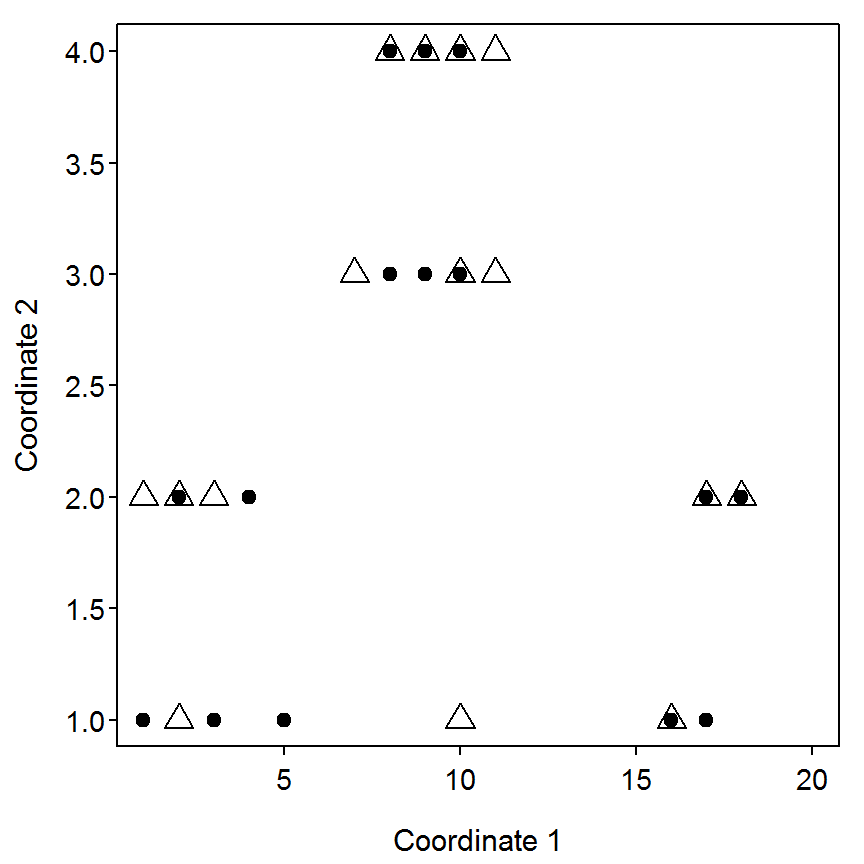> plot(coord2~coord1,data=d5Bv,pch=2,cex=1.5,xlab="Coordinate 1",ylab="Coordinate 2",
xlim=c(1,20),ylim=c(1,4))
> points(coord2~coord1,data=d5Bf,pch=19,cex=1)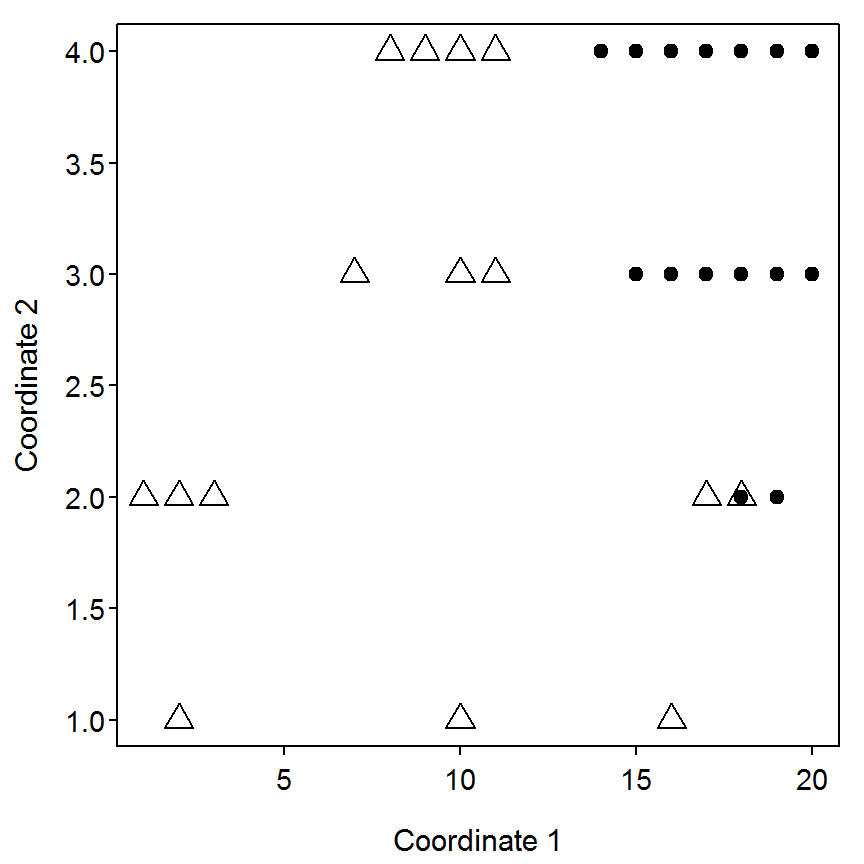### 11.5.3 Scenario A (“Same”" Distributions)

The ks2d2() function (from the FSA package) is used to perform the two-dimensional Kolmogorov-Smirnov test. This function requires the two vectors of coordinates for one group of data as the first two arguments and the two vectors of coordinates for the second group of data as the next two (third and fourth) arguments. A plot depicting the maximum is observed by submitting the saved ks2d2 argument to plot().

> ( scenA <- ks2d2(d5Av$coord1,d5Av$coord2,d5Af$coord1,d5Af$coord2) )
Two Dimensional Kolmogorov-Smirnov Test - THESE RESULTS ARE EXPERIMENTAL AT THIS POINT!!!

Maximum for first coordinate set as origins was 0.1333 for observation # 3 8 10 13 15
Maximum for second coordinate set as origins was 0.1333 for observation # 2 10 11 12 14

Resulting in a test statistic (D) of 0.1333 
> plot(scenA)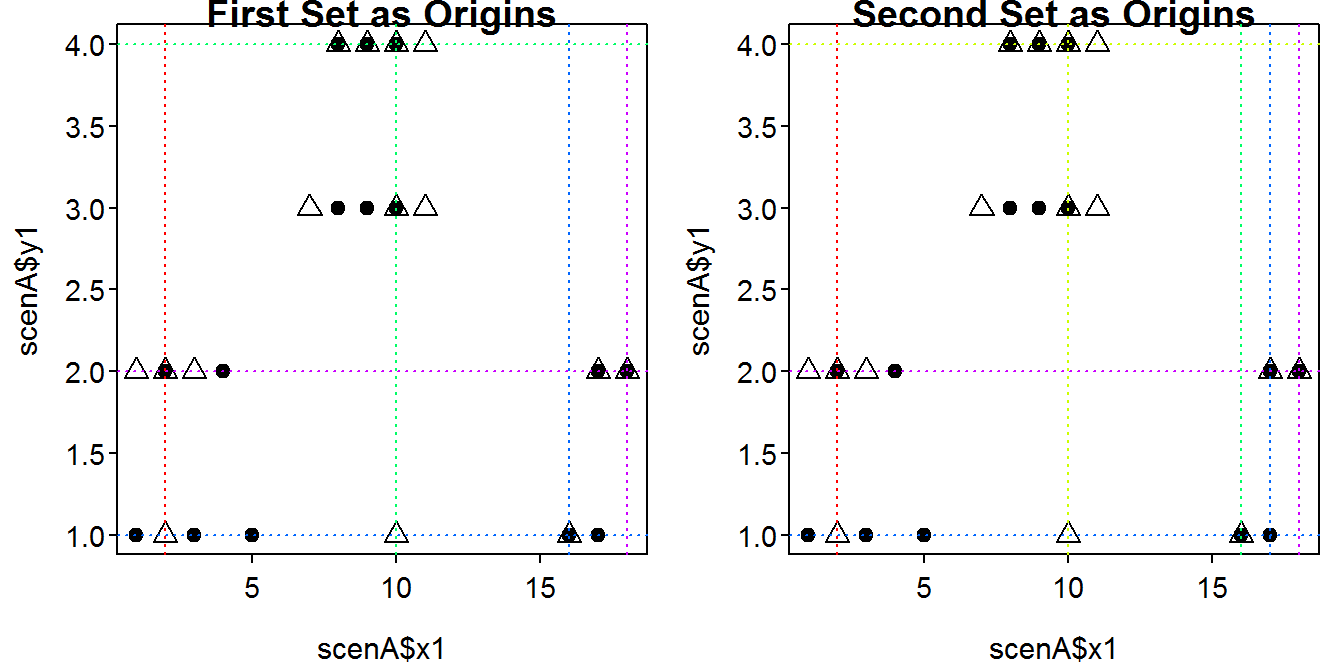The resampling p-value is computed with ks2d2p() which requires the saved ks2d2 argument and the number of resamples to use in the B= argument. A plot of the density of resamples is constructed by submitting the saved ks2dp object to plot().

> ( scenAp <- ks2d2p(scenA,B=1000) )
Two-Sample Two-Dimensional Kolmogorov-Smirnov Test p-value - THESE RESULTS ARE EXPERIMENTAL AT THIS POINT!!!
Used 'resample' method B= 1000 times
D= 0.1333 , p-value = 0.981 
> plot(scenAp)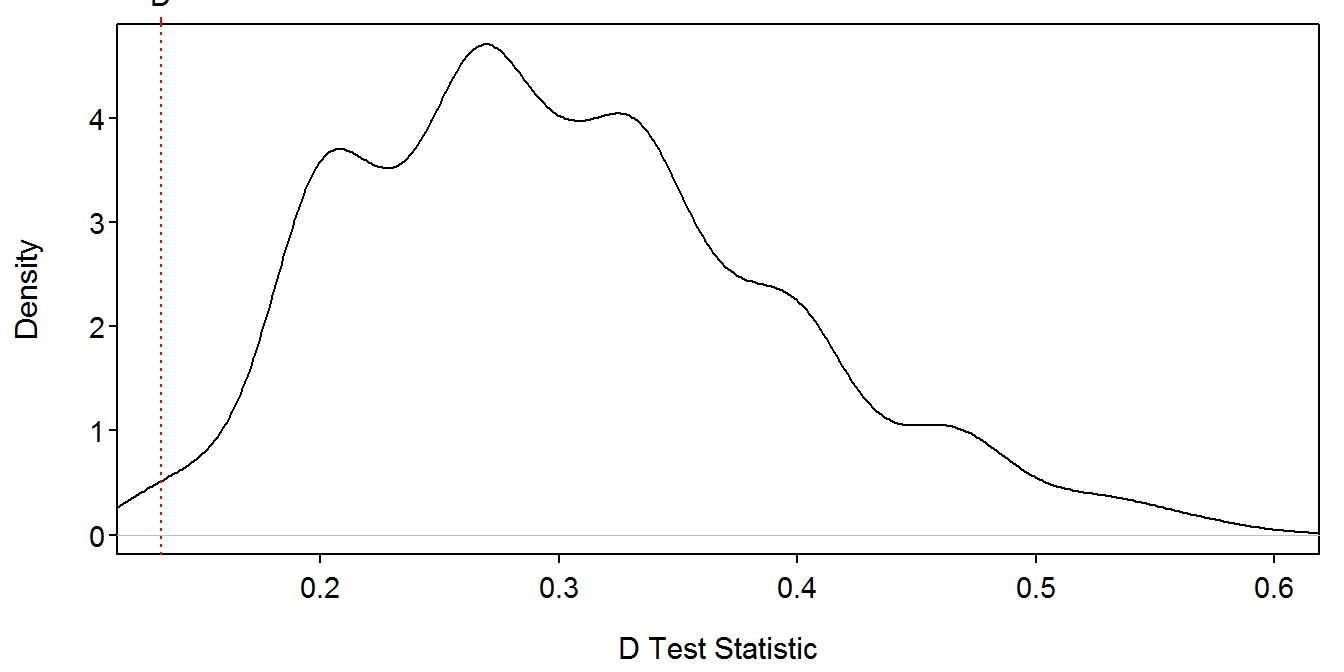### 11.5.4 Scenario B (“Different” Distributions)

Similar results for scenario B are shown below.

> ( scenB <- ks2d2(d5Bv$coord1,d5Bv$coord2,d5Bf$coord1,d5Bf$coord2) )
Two Dimensional Kolmogorov-Smirnov Test - THESE RESULTS ARE EXPERIMENTAL AT THIS POINT!!!

Maximum for first coordinate set as origins was 0.7333 for observation # 8
Maximum for second coordinate set as origins was 0.5333 for observation # 1 3

Resulting in a test statistic (D) of 0.6333 
> plot(scenB)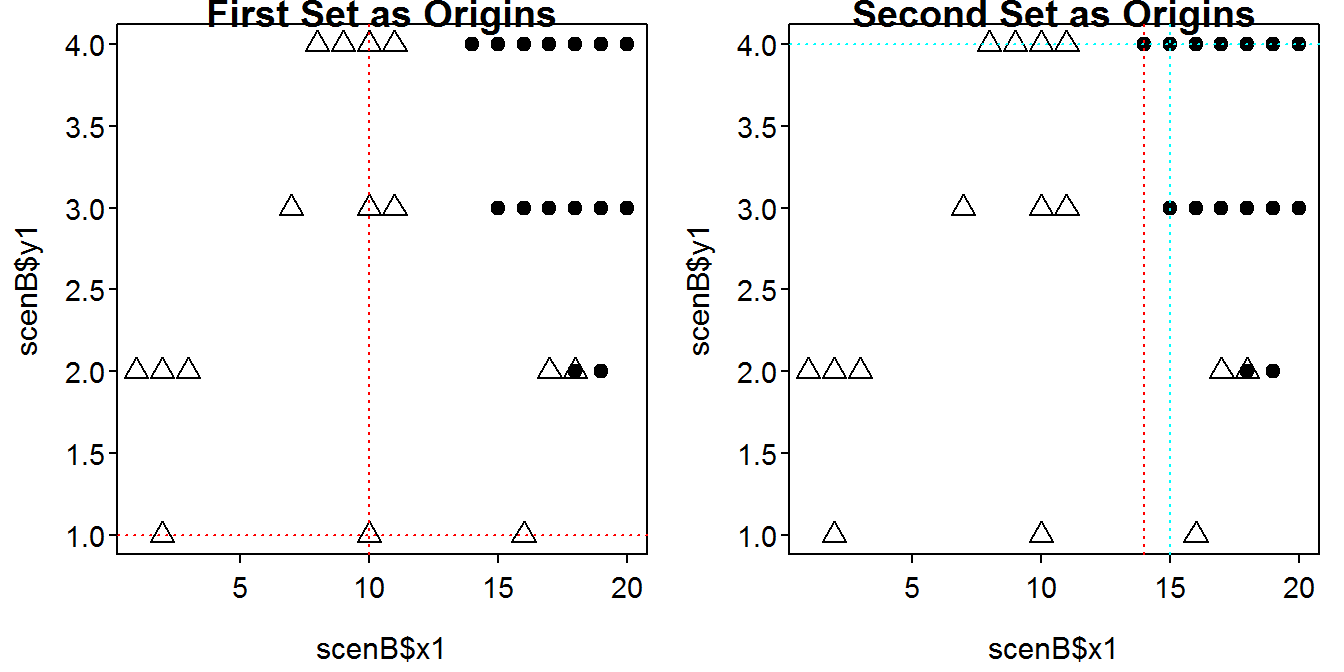> ( scenBp <- ks2d2p(scenB,B=1000) )
Two-Sample Two-Dimensional Kolmogorov-Smirnov Test p-value - THESE RESULTS ARE EXPERIMENTAL AT THIS POINT!!!
Used 'resample' method B= 1000 times
D= 0.6333 , p-value = 0.002 
> plot(scenBp)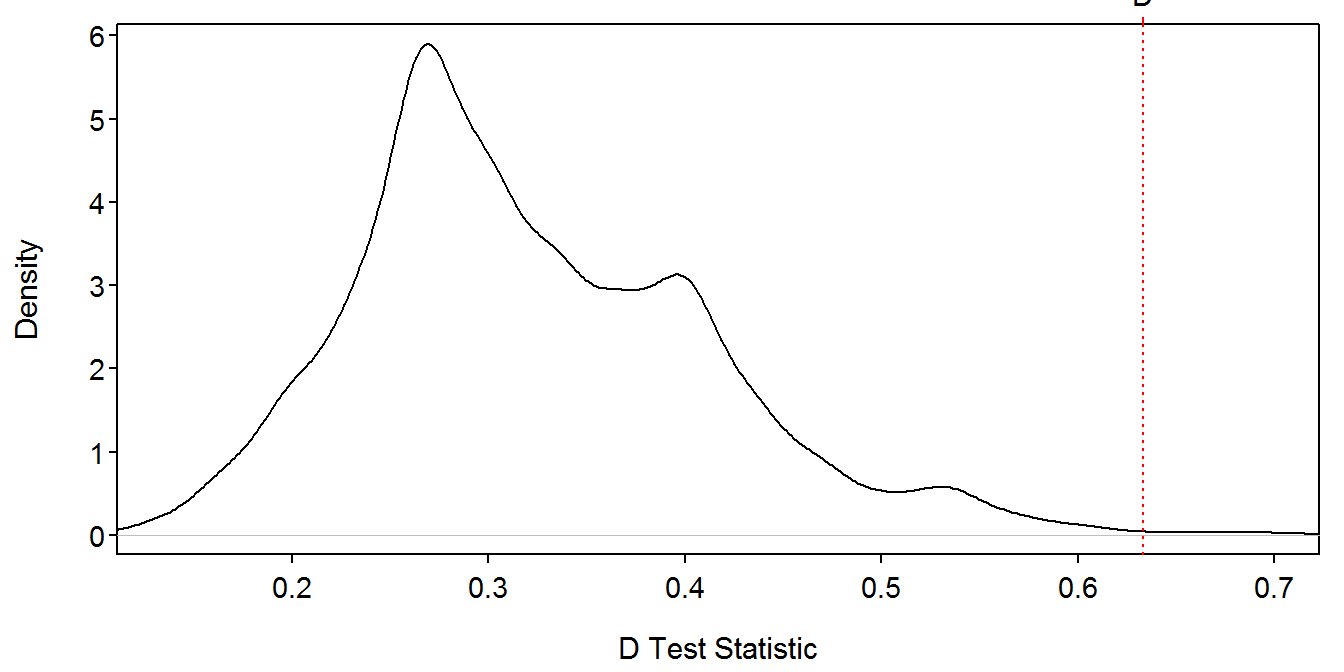## 11.6 Determining Diet Patterns in Diet Data with Analysis of Covariance (ANCOVA)

We often want to determine if diel patterns in diet data occur. This has important implications for designing sampling protocols and interpreting diet data. One way to determine whether diel variation in feeding occurs is by sampling fish during different times of the day.

### 11.6.1 Preparing Data

The Box11_6.txt data file is read and the structure is observed.

> d6 <- read.table("data/Box11_6.txt",header=TRUE)
> str(d6)
'data.frame':   36 obs. of  3 variables:
$time : Factor w/ 3 levels "evening","morning",..: 2 2 2 2 2 2 2 2 2 2 ...$ length: int  23 26 34 38 41 44 52 54 67 68 ...
$diet : int 110 90 75 100 130 80 210 130 140 300 ... The data provided on the book CD appears to faithfully reproduce the results shown in the figure in the box. However, the stomach contents weight variable appears to have been divided by 100 to produce the ANOVA table shown in the box. I will use the stomach content weight data as provided on the CD and NOT divided by 100. The interpretations are exactly the same with both sets of data; however, the exact SS and MS values in the output are different by a constant amount. ### 11.6.2 Quick Exploration A scatterplot of stomach contents weight on predator length separated by time of day can be constructed with plot() and points() similar to what was shown above. > # base empty figure > plot(diet~length,data=d6,type="n",xlab="Predator Length (mm)", ylab="Stomach Contents Weight (mg)") > # now add points > points(diet~length,data=d6,subset=time=="evening",pch=19,col="black") > points(diet~length,data=d6,subset=time=="morning",pch=1,col="blue") > points(diet~length,data=d6,subset=time=="noon",pch=3,col="red") > legend("topleft",legend=c("evening","morning","noon"),pch=c(19,1,3),col=c("black","blue","red"))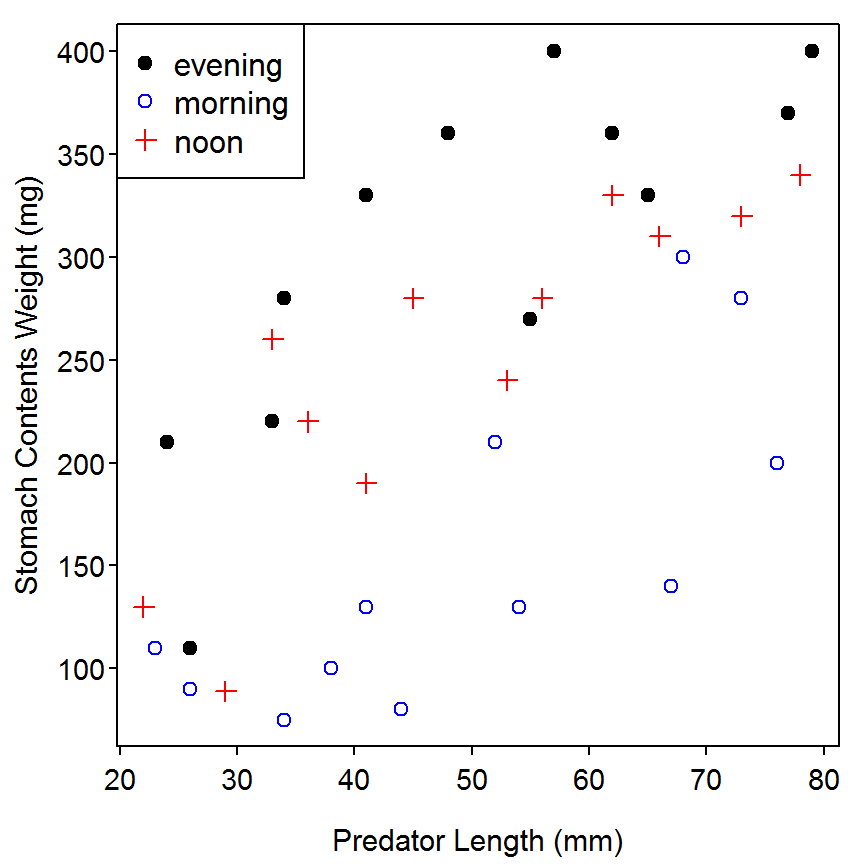### 11.6.3 ANCOVA Results I The ANCOVA is fit with lm() using a formula of the form response~quantitative*factor, where quantitative represents the quantitative covariate variable and factor represents the categorical group factor variable. The right-hand-side of this formula is short-hand to tell R to fit the two main effects plus the interaction term. The type-III ANOVA table is constructed by submitting the lm object to Anova() (from the car package) with the type="III" argument. As described in the box, the high p-value for the interaction term ($$p=0.8560$$) suggests that the slopes among the three times are statistically equal. > lm1 <- lm(diet~length*time,data=d6) > Anova(lm1,type="III")  Sum Sq Df F value Pr(>F) (Intercept) 17979 1 7.2065 0.01171 length 55455 1 22.2279 5.209e-05 time 9843 2 1.9727 0.15672 length:time 780 2 0.1563 0.85602 Residuals 74845 30 Total 36 158902  The model with the interaction term dropped is fit and summarized below. These results suggest that the intercept, and, thus, the predicted stomach content weights at all predator lengths, is different among the three times ($$p<0.00005$$) and that the relationship between stomach contents weight and predator length is significantly positive ($$p<0.00005$$). > lm2 <- lm(diet~length+time,data=d6) > Anova(lm2,type="III")  Sum Sq Df F value Pr(>F) (Intercept) 48951 1 20.713 7.287e-05 length 144309 1 61.063 6.501e-09 time 135397 2 28.646 7.403e-08 Residuals 75625 32 Total 36 404281  A visual of the model fit is obtained by submitting the saved lm object to fitPlot(), from the FSA package. > fitPlot(lm2,xlab="Predator Length (mm)",ylab="Stomach Contents Weight (mg)",legend="topleft")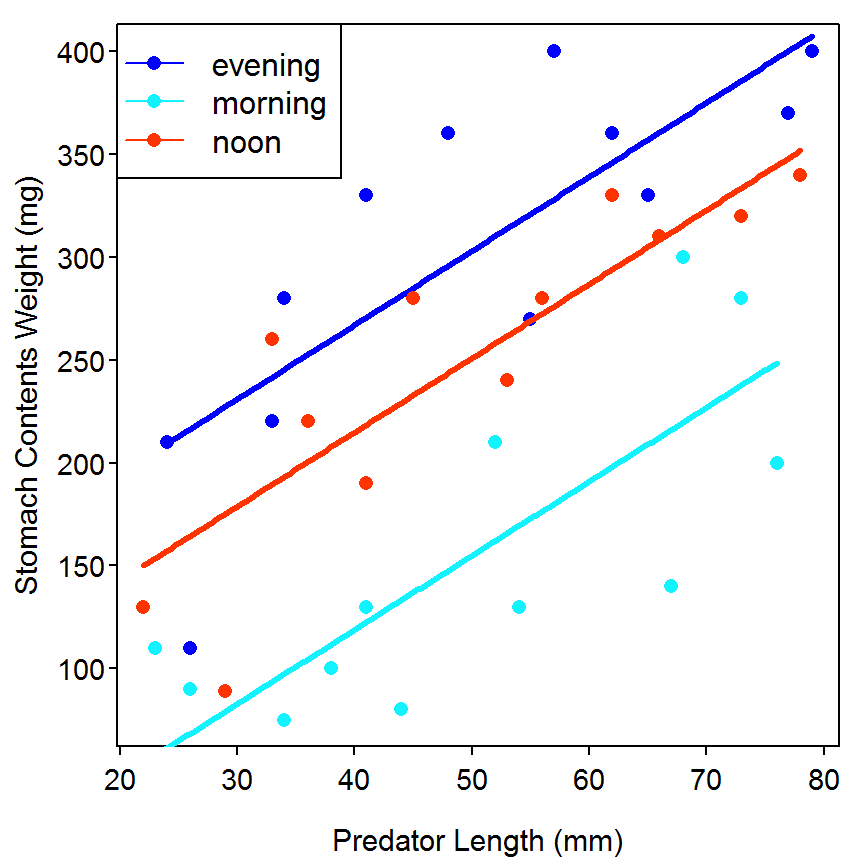## 11.7 Comparing Diet Data from Different Locations or Times with Multivariate Analysis of Variance (MANOVA) Because of the multivariate nature of diet data, we are often interested in determining whether diet composition differs among fishes sampled from different locations or at different times. When diet data are measured as prey mass (or volume), MANOVA can be useful for testing an overall location (or time) effect. ### 11.7.1 Preparing Data The Box11_7.txt data file is read and the structure is observed. > d7 <- read.table("data/Box11_7.txt",header=TRUE) > str(d7) 'data.frame': 15 obs. of 6 variables:$ lake : Factor w/ 3 levels "LakeA","LakeB",..: 1 1 1 1 1 2 2 2 2 2 ...
$fish : int 1 2 3 4 5 6 7 8 9 10 ...$ chiro: num  0.12 0.09 0.12 0.26 0.27 0.49 0.36 0.34 0.42 0.57 ...
$amph : num 0.35 0.22 0.35 0.38 0.29 0.01 0.04 0.05 0.03 0.11 ...$ odon : num  0.44 0.63 0.5 0.22 0.27 0.38 0.59 0.57 0.24 0.21 ...
$zoo : num 0.09 0.06 0.03 0.14 0.17 0.12 0.01 0.04 0.31 0.11 ... ### 11.7.2 Fitting the Model A multivariate ANOVA (MANOVA) is performed in R with manova(), from the MASS package. This function requires a model formula as the first argument where the left-hand-side of the formula is a matrix containing just the response variables and the right-hand-side contains the group factor variables. In most cases the response variables will need to be extracted from a data frame, wrapped in as.matrix() to force them into a matrix object, and saved to a new object as shown below. One might wonder why the four prey items are not included in this matrix. As the data is the proportional composition of each prey item in each fish, the four items necessarily sum to 1. This means that the value of each prey item can be perfectly derived by knowing the values of the other three prey items. Models cannot be fit in R when one of the values is a perfect linear combination of the other values. Thus, the prey items will be examined three at a time in this example. > Y1 <- as.matrix(d7[,c("chiro","amph","odon")]) The response matrix and the lake explanatory variable are then used to form the formula in manova(). The four test statistics shown in the first table on page 498 are extracted individually from the saved manova() object with summary() and various versions of the test= argument as illustated below. > man1 <- manova(Y1~d7$lake)
> summary(man1,test="Wilks")
          Df    Wilks approx F num Df den Df   Pr(>F)
d7$lake 2 0.079047 8.5226 6 20 0.000113 Residuals 12  > summary(man1,test="Pillai")  Df Pillai approx F num Df den Df Pr(>F) d7$lake    2 0.92593   3.1609      6     22 0.02169
Residuals 12                                       
> summary(man1,test="Hotelling-Lawley")
          Df Hotelling-Lawley approx F num Df den Df    Pr(>F)
d7$lake 2 11.588 17.382 6 18 1.343e-06 Residuals 12  > summary(man1,test="Roy")  Df Roy approx F num Df den Df Pr(>F) d7$lake    2 11.582   42.469      3     11 2.436e-06
Residuals 12                                        

Three of the four results from the individual response variable ANOVAs shown in the last table on page 498 are extracted by including the saved manova object to summary.aov(). The fourth individual ANOVA is not extracted above because the fourth prey item could not be included in the response vector as described previously.

> summary.aov(man1)
 Response chiro :
Df  Sum Sq  Mean Sq F value    Pr(>F)
d7$lake 2 0.37925 0.189627 31.378 1.711e-05 Residuals 12 0.07252 0.006043 Total 14 0.45177 Response amph : Df Sum Sq Mean Sq F value Pr(>F) d7$lake      2 0.36321 0.181607  20.979 0.000121
Residuals   12 0.10388 0.008657
Total       14 0.46709

Response odon :
Df  Sum Sq Mean Sq F value Pr(>F)
d7$lake 2 0.00724 0.00362 0.1329 0.8768 Residuals 12 0.32676 0.02723 Total 14 0.33400  The fourth individual ANOVA can be obtained by creating a different response vector and repeating the process above. > Y2 <- as.matrix(d7[,c("amph","odon","zoo")]) # put zoo in to get last indiv ANOVAs > man2 <- manova(Y2~d7$lake)
> summary(man2,test="Wilks")                   # show that the results are the same
          Df    Wilks approx F num Df den Df   Pr(>F)
d7$lake 2 0.079047 8.5226 6 20 0.000113 Residuals 12  > summary.aov(man2)  Response amph : Df Sum Sq Mean Sq F value Pr(>F) d7$lake      2 0.36321 0.181607  20.979 0.000121
Residuals   12 0.10388 0.008657
Total       14 0.46709

Response odon :
Df  Sum Sq Mean Sq F value Pr(>F)
d7$lake 2 0.00724 0.00362 0.1329 0.8768 Residuals 12 0.32676 0.02723 Total 14 0.33400 Response zoo : Df Sum Sq Mean Sq F value Pr(>F) d7$lake      2 0.00400 0.00200  0.3407 0.7179
Residuals   12 0.07044 0.00587
Total       14 0.07444                       

### 11.7.3 Sums-of-Squares and Cross-Products Matrices

The SAS code in the box implies that the authors printed the sums-of-squares and cross-products matrices for the error and treatments (the printe and printh commands), though these results are not shown in the box. The commands below would print these matrices in R.

> ( n <- nrow(Y1) )
 15
> ( E1 <- (n-1)*cov(man1$residuals) ) # Error SSCP; diagonals are indiv SSerror  chiro amph odon chiro 0.07252 0.01630 -0.11536 amph 0.01630 0.10388 -0.11730 odon -0.11536 -0.11730 0.32676 > ( H1 <- (n-1)*cov(man1$fitted.values) ) # Treatment SSCP; diagonals are indiv SStreats
           chiro       amph     odon
chiro  0.3792533 -0.3708133 -0.04644
amph  -0.3708133  0.3632133  0.04440
odon  -0.0464400  0.0444000  0.00724

## 11.8 Testing Prey Counts with Multiway Contingency Table Analysis

When diet data are measured as prey counts, multiway contingency table analysis can be used to test for treatment effects.

### 11.8.1 Preparing Data

The Box11_8.txt data file is read and the file is observed.

> d8 <- read.table("data/Box11_8.txt",header=TRUE)
> d8
          prey    stage  habitat number
5    amphipods juvenile littoral     19
6  chironomids juvenile littoral     43
7     mayflies juvenile littoral      4
8    ostracods juvenile littoral      6
13   amphipods juvenile  pelagic      1
14 chironomids juvenile  pelagic      8
15    mayflies juvenile  pelagic      5
16   ostracods juvenile  pelagic      4

NEEDS WORK HERE

## 11.9 Exploring Diet Data with Principal Components Analysis

Traditional multivariate techniques, such as PCA, can be constrained by the compositional nature of diet data in so much as the row sums must equal one. Log-ratio analysis, such as %PCA (see text), is performed on the logarithm of proportions and can be useful for exploring individual variation in diet data. For values equal to zero, very small numbers (e.g., 0.00001) are entered prior to analysis as recommended by Aitchison (1983). A %PCA analysis was performed on the diet composition data given in Table 11.2. The first two components accounted for 94% (%PC1=60%, %PC2=34%) of the total variation in diet data.

### 11.9.1 Preparing Data

The Box11_9.txt data file is read and the structure is observed. The proportion by weight of each prey species is computed and added to the data frame by dividing the weight of the prey by the total stomach contents weight. The natural logarithms for each of the proportions is then computed but, first, to “adjust” for zeroes in the data, a small amount was added to each.

> d9 <- read.table("data/Box11_9.txt",header=TRUE)
> str(d9)
'data.frame':   10 obs. of  7 variables:
$bluegill: Factor w/ 10 levels "A","B","C","D",..: 1 2 3 4 5 6 7 8 9 10$ wt      : int  150 91 99 123 210 102 124 199 101 111
$amph : num 0.3 0.09 0.11 0.03 0 0.22 0 0.015 0.45 0.26$ lfish   : num  0.24 0 0 0 0.12 0 0 0.12 0 0.36
$dipt : num 0.02 0.018 0.024 0.052 0.001 0.003 0.006 0.003 0.015 0.054$ mayfly  : num  0.016 0.008 0.032 0.04 0.024 0.016 0.056 0.032 0.032 0
$ttlwt : num 0.576 0.116 0.166 0.122 0.145 0.239 0.062 0.17 0.497 0.674 > # add proportions > d9$pAmph <- d9$amph/d9$ttlwt
> d9$pLFish <- d9$lfish/d9$ttlwt > d9$pDipt <- d9$dipt/d9$ttlwt
> d9$pMayfly <- d9$mayfly/d9$ttlwt > # add logs of proportions > amt <- 0.00001 # small amount to adjust for zeroes > d9$lpAmph <- log(d9$pAmph+amt) > d9$lpLFish <- log(d9$pLFish+amt) > d9$lpDipt <- log(d9$pDipt+amt) > d9$lpMayfly <- log(d9$pMayfly+amt) > # what does it all look like now > str(d9) 'data.frame': 10 obs. of 15 variables:$ bluegill: Factor w/ 10 levels "A","B","C","D",..: 1 2 3 4 5 6 7 8 9 10
$wt : int 150 91 99 123 210 102 124 199 101 111$ amph    : num  0.3 0.09 0.11 0.03 0 0.22 0 0.015 0.45 0.26
$lfish : num 0.24 0 0 0 0.12 0 0 0.12 0 0.36$ dipt    : num  0.02 0.018 0.024 0.052 0.001 0.003 0.006 0.003 0.015 0.054
$mayfly : num 0.016 0.008 0.032 0.04 0.024 0.016 0.056 0.032 0.032 0$ ttlwt   : num  0.576 0.116 0.166 0.122 0.145 0.239 0.062 0.17 0.497 0.674
$pAmph : num 0.521 0.776 0.663 0.246 0 ...$ pLFish  : num  0.417 0 0 0 0.828 ...
$pDipt : num 0.0347 0.1552 0.1446 0.4262 0.0069 ...$ pMayfly : num  0.0278 0.069 0.1928 0.3279 0.1655 ...
$lpAmph : num -0.652 -0.254 -0.411 -1.403 -11.513 ...$ lpLFish : num  -0.875 -11.513 -11.513 -11.513 -0.189 ...
$lpDipt : num -3.36 -1.863 -1.934 -0.853 -4.975 ...$ lpMayfly: num  -3.58 -2.67 -1.65 -1.12 -1.8 ...
> headtail(d9)
   bluegill  wt  amph lfish  dipt mayfly ttlwt      pAmph    pLFish      pDipt
1         A 150 0.300  0.24 0.020  0.016 0.576 0.52083333 0.4166667 0.03472222
2         B  91 0.090  0.00 0.018  0.008 0.116 0.77586207 0.0000000 0.15517241
3         C  99 0.110  0.00 0.024  0.032 0.166 0.66265060 0.0000000 0.14457831
8         H 199 0.015  0.12 0.003  0.032 0.170 0.08823529 0.7058824 0.01764706
9         I 101 0.450  0.00 0.015  0.032 0.497 0.90543260 0.0000000 0.03018109
10        J 111 0.260  0.36 0.054  0.000 0.674 0.38575668 0.5341246 0.08011869
pMayfly     lpAmph     lpLFish    lpDipt   lpMayfly
1  0.02777778 -0.6523060  -0.8754447 -3.360087  -3.583159
2  0.06896552 -0.2537676 -11.5129255 -1.863154  -2.674004
3  0.19277108 -0.4114923 -11.5129255 -1.933865  -1.646200
8  0.18823529 -2.4276349  -0.3482925 -4.036620  -1.670009
9  0.06438632 -0.0993314 -11.5129255 -3.500209  -2.742699
10 0.00000000 -0.9525226  -0.6271074 -2.524121 -11.512925

Finally, the logged proportion data was isolated to make entries to functions used below easier.

> d9a <- d9[,c("bluegill","lpAmph","lpLFish","lpDipt","lpMayfly")]
> str(d9a)
'data.frame':   10 obs. of  5 variables:
$bluegill: Factor w/ 10 levels "A","B","C","D",..: 1 2 3 4 5 6 7 8 9 10$ lpAmph  : num  -0.652 -0.254 -0.411 -1.403 -11.513 ...
$lpLFish : num -0.875 -11.513 -11.513 -11.513 -0.189 ...$ lpDipt  : num  -3.36 -1.863 -1.934 -0.853 -4.975 ...
$lpMayfly: num -3.58 -2.67 -1.65 -1.12 -1.8 ... ### 11.9.2 Principal Components Analysis The principal components analysis (PCA) is computed with princomp() which requires the matrix or data frame of just the variables to be analyzed as the first argument. A summary of the PCA is obtained by submitting the saved princomp object to summary(). These results show that 90.6% of the overall variability is explained by the first two principal components. The explained variances are also illustrated with a so-called scree plot constructed by submitting the saved princomp object to screeplot(). > pc1 <- princomp(d9a[,-1]) # ignore first column of bluegill IDs > summary(pc1) Importance of components: Comp.1 Comp.2 Comp.3 Comp.4 Standard deviation 5.7065907 4.5140807 2.13182292 0.98003010 Proportion of Variance 0.5571723 0.3486380 0.07775679 0.01643293 Cumulative Proportion 0.5571723 0.9058103 0.98356707 1.00000000 > screeplot(pc1,type="lines")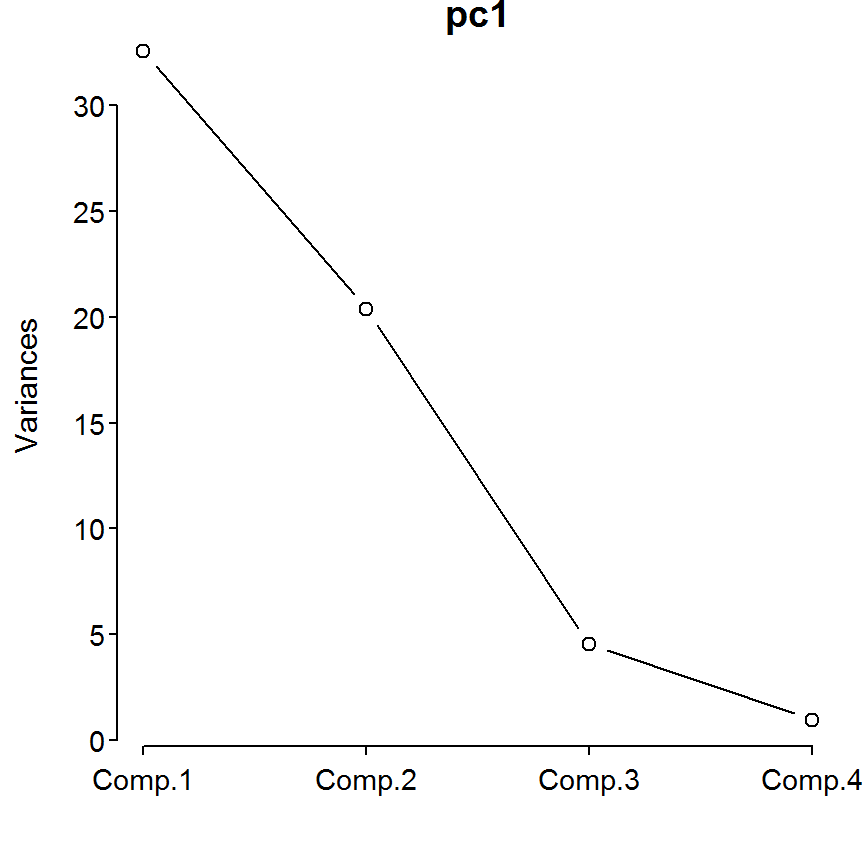The loadings of each principal component are extracted from the princomp object with loadings(). The top portion of this output shows that the first PC is largely inversely related to the proportion of larval fish in the diet and the second PC is mostly positively related to the proportion of Amphipods and somewhat negatively related to the proportion of mayflies. These loadings, along with the relative position of the individual fish, are illustrated using biplot() with the princomp object as the first argument and a vector of labels for the individual fish in the xlabs= argument. > loadings(pc1)  Loadings: Comp.1 Comp.2 Comp.3 Comp.4 lpAmph 0.246 0.893 -0.377 lpLFish -0.932 0.131 -0.302 0.151 lpDipt 0.112 0.163 0.979 lpMayfly 0.240 -0.429 -0.860 0.136 Comp.1 Comp.2 Comp.3 Comp.4 SS loadings 1.00 1.00 1.00 1.00 Proportion Var 0.25 0.25 0.25 0.25 Cumulative Var 0.25 0.50 0.75 1.00 > biplot(pc1,xlabs=d9a$bluegill)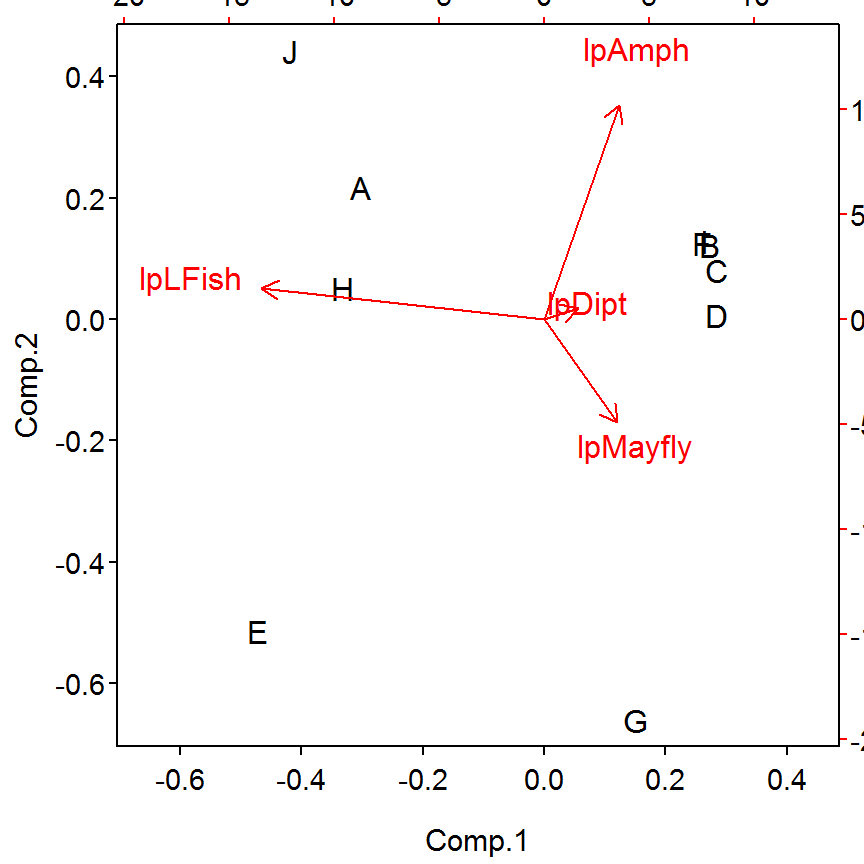### 11.9.3 Correlation Analysis

The correlation between fish weight and the first two principal components is computed with rcorr(), from the Hmisc package. Note that rcorr() requires a matrix as its first argument; thus, the fish weights and the first two principal components must be “column-bound” together to form a matrix. These results show that there is a significant negative correlation between the first PC and Bluegill weight. Thus, larger Bluegills consume greater amounts of larval fish (remember that more negative values of PC1 means more larval fish as the proportion of larval fish and the PC1 are negatively related). This relationship is visualized with the scatterplot below.

> rcorr(cbind(d9$wt,pc1$scores[,1:2]))
             Comp.1 Comp.2
1.00  -0.76  -0.41
Comp.1 -0.76   1.00   0.00
Comp.2 -0.41   0.00   1.00

n= 10

P
Comp.1 Comp.2
0.0111 0.2370
Comp.1 0.0111        1.0000
Comp.2 0.2370 1.0000       
> plot(pc1$scores[,1]~d9$wt,pch=19,xlab="Bluegill Weight",ylab="PC1")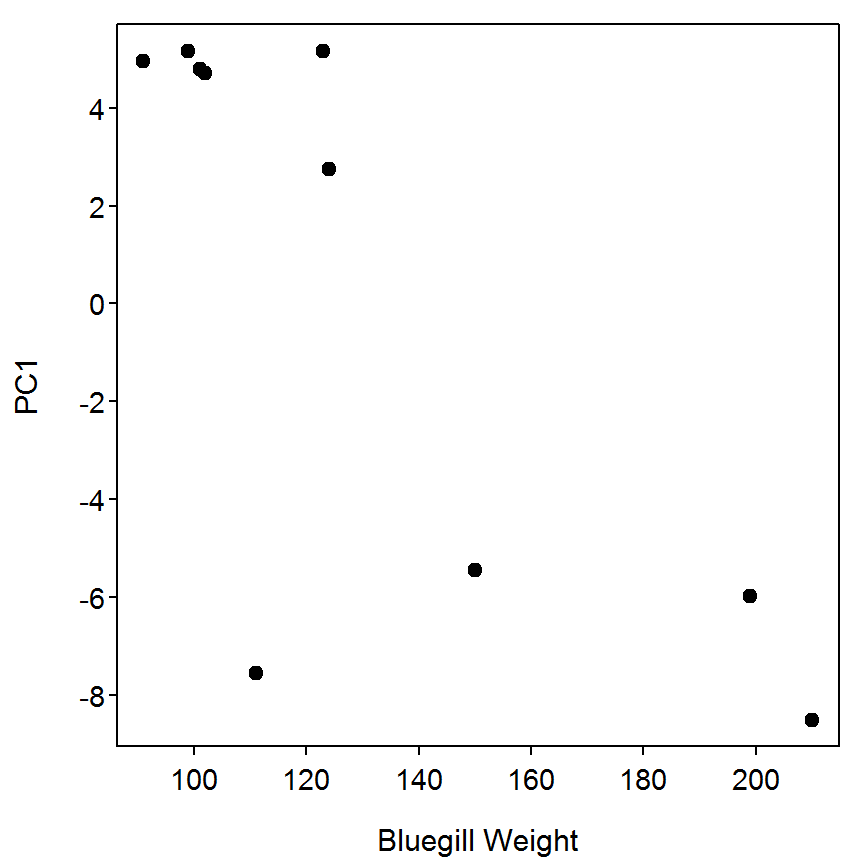### 11.9.4 Ogle Comment

The PCA results from R do not match the PCA results shown in the box. Generally, PCA results often differ between softwares as the eigenvectors are not unique and can be rotated. There is not enough information in the box to more fully compare these results.

## 11.10 Assessing Prey Preference

Differences in prey selectively provide important insight about foraging patterns of fishes. In many cases, these type of data are collected under controlled, experimental settings in which changes in the absolute abundance of prey can be accurately determined.

Catalano et al. (2001) examined the effects of tag color on vulnerability to predation. Age-0 Bluegills (Lepomis macrochirus) were marked with either brightly colored fluorescent tags or cryptic tags and then exposed to Largemouth Bass (Micropterus salmoides) predators in a series of tank experiments. Manly’s alpha was calculated using the equation for variable prey populations (equation 11.8 in text).

### 11.10.1 Ogle Comment

Equation 11.8 in the text for computing the Manly-Chesson index appears to be incorrect. The calculations on the companion CD are different, with the values in the sum in the denominator being logged, which also appears to be incorrect. Most references (e.g., see first page of Chesson (1983)) show that the denominator in equation 11.8 is correct but the numerator should NOT be logged as was done on the companion CD. I will use the version where neither the numerator nor the denominator contain any logs.

### 11.10.2 Preparing Data

The Box11_10.txt data file is read and the structure is observed. The proportion remaining in each trial and tag color is computed and appended to the data frame.

> d10 <- read.table("data/Box11_10.txt",header=TRUE)
> str(d10)
'data.frame':   6 obs. of  4 variables:
$trial : int 1 1 2 2 3 3$ tag.color : Factor w/ 2 levels "Bright","Cryptic": 1 2 1 2 1 2
$prey.init : int 120 60 96 48 150 75$ prey.final: int  22 21 26 15 64 35

> resids <- lm1$residuals > mean(abs(resids))  0.07221796 ### 11.11.5 Using Provided Equation The authors’ provided the following equation for the relationship between trophic position and Walleye length: TP=2.797+0.001445*LEN. This equation can be added to the previous plot with abline() to see how it differs. > fitPlot(lm1,xlab="Walleye Length (mm)",ylab="Trophic Position",main="") > abline(coef=c(2.797,0.001445),lwd=2,lty=3,col="red")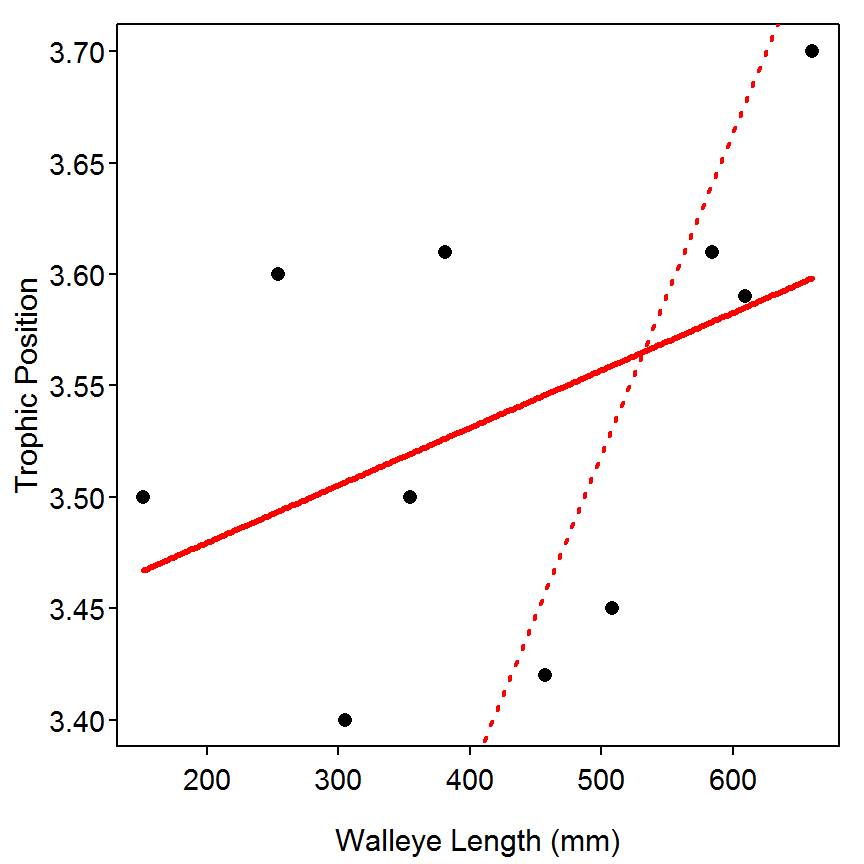The summarization of the residuals is performed by first subtracting the predicted length from the authors provided equation from the observed trophic positions. > resids2 <- d11$tp-(2.797+0.001445*d11\$len)
> mean(abs(resids2))
 0.1821095

Reproducibility Information
Compiled Date: Thu May 14 2015
Compiled Time: 6:25:13 PM
Code Execution Time: 4.63 s

R Version: R version 3.2.0 (2015-04-16)
System: Windows, i386-w64-mingw32/i386 (32-bit)
Base Packages: base, datasets, graphics, grDevices, grid, methods, stats,
utils
Required Packages: FSA, car, ggplot2, Hmisc, lattice, MASS, quantreg and
their dependencies (acepack, cluster, digest, dplyr, foreign, Formula,
gdata, gplots, graphics, grDevices, grid, gridExtra, gtable, knitr,
latticeExtra, lmtest, methods, mgcv, multcomp, nnet, pbkrtest, plotrix,
plyr, proto, relax, reshape2, rpart, scales, sciplot, SparseM, stats,
survival, utils)
Other Packages: car_2.0-25, Formula_1.2-1, FSA_0.6.13, FSAdata_0.1.9,
ggplot2_1.0.1, Hmisc_3.16-0, knitr_1.10.5, lattice_0.20-31,
MASS_7.3-40, multcomp_1.4-0, mvtnorm_1.0-2, NCStats_0.4.3,
quantreg_5.11, rmarkdown_0.6.1, SparseM_1.6, survival_2.38-1,
TH.data_1.0-6
caTools_1.17.1, cluster_2.0.1, codetools_0.2-11, colorspace_1.2-6,
DBI_0.3.1, digest_0.6.8, dplyr_0.4.1, evaluate_0.7, foreign_0.8-63,
formatR_1.2, gdata_2.16.1, gplots_2.17.0, gridExtra_0.9.1,
gtable_0.1.2, gtools_3.4.2, highr_0.5, htmltools_0.2.6,
KernSmooth_2.23-14, labeling_0.3, latticeExtra_0.6-26, lme4_1.1-7,
lmtest_0.9-33, magrittr_1.5, Matrix_1.2-0, mgcv_1.8-6, minqa_1.2.4,
munsell_0.4.2, nlme_3.1-120, nloptr_1.0.4, nnet_7.3-9, parallel_3.2.0,
pbkrtest_0.4-2, plotrix_3.5-11, plyr_1.8.2, proto_0.3-10,
RColorBrewer_1.1-2, Rcpp_0.11.6, relax_1.3.15, reshape2_1.4.1,
rpart_4.1-9, sandwich_2.3-3, scales_0.2.4, sciplot_1.1-0,
splines_3.2.0, stringi_0.4-1, stringr_1.0.0, tools_3.2.0, yaml_2.1.13,
zoo_1.7-12

## References

Aitchison, J. 1983. Principal components analysis of compositional data. Biometrika 70:57–65.

Cade, B. S., and J. D. Richards. 2000. User manual for Blossom Statistical Software. Midcontinential Ecological Science Center, Us. Geological Survey, Fort Collins, Collorado.

Catalano, M. J., S. R. Chipps, M. A. Bouchard, and D. H. Wahl. 2001. Evaluation of injectable flourescent tags for centrarchid fishs: Mark retention rate and effects on vulnerability to predation. North American Journal of Fisheries Management 21:911–917.

Chesson, J. 1983. The estimation and analysis of preference and its relationship to foraging models. Ecology 64:1297–1304.

Fortin, M., and J. Gurevitch. 1993. Design and analysis of ecological experiments. Pages 342–359 in S. M. Scheiner and J. Gurevitch, editors. Chapman; Hall, New York.

Garvey, J. E., E. A. Marschall, and R. A. Wright. 1998. From star charts to stoneflies: Detecting relationship in continuous bivariate data. Ecology 79:442–447.

Scharf, F. S., F. Juanes, and M. Sutherland. 1998. Estimating piscine prey size from partial remains: Testing for shifts in foraging mode by juvenile bluefish. Ecology 79:448–460.

Vander Zanden, M. J., B. J. Shuter, N. P. Lester, and J. B. Rasmussen. 2000. Within- and among-population variation in the trophic position of pelagic predator, lake trout (Salvelinus namaycush). Canadian Journal of Fisheries and Aquatic Sciences 57:725–731.